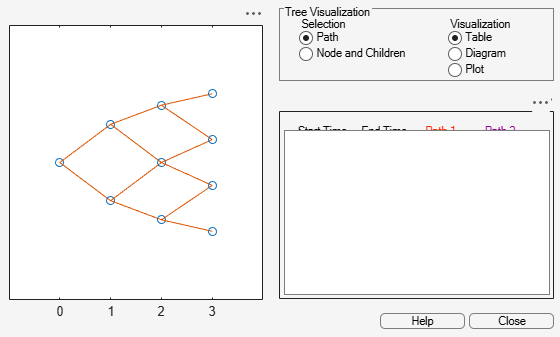# treeshape

Shape of recombining binomial tree

## Syntax

``[NumLevels,NumPos,IsPriceTree] = treeshape(Tree)``

## Description

example

````[NumLevels,NumPos,IsPriceTree] = treeshape(Tree)` returns information on a recombining binomial tree's shape.```

## Examples

collapse all

This example shows how to obtain information on a recombining binomial tree's shape.

`load deriv.mat; `

With `treeviewer` you can see the general shape of the BDT interest-rate tree.

`treeviewer(BDTTree)`Use `treeshape` to display the shape of the binomial tree.

`[NumLevels, NumPos, IsPriceTree] = treeshape(BDTTree.FwdTree) `
```NumLevels = 4 ```
```NumPos = 1×4 1 1 1 1 ```
```IsPriceTree = logical 0 ```

## Input Arguments

collapse all

Recombining binomial tree, specified as a struct that is created using one of the following functions:

Data Types: `struct`

## Output Arguments

collapse all

Number of time levels of tree, returned as a numeric.

Length of the state vectors in each level, returned as a `1`-by-`NUMLEVELS` vector.

Indicates if final horizontal branch is present in the tree, returned as a Boolean.

## Version History

Introduced before R2006a